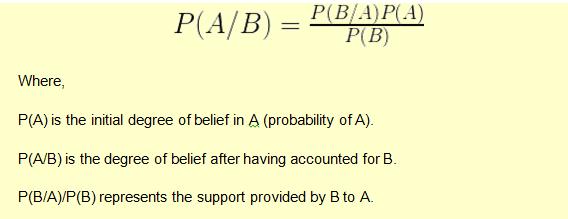# Bayes’ Theorem

Bayes’ Theorem formula, also known as Bayes’ Law, or Bayes’ Rule, is an intuitive idea. We adjust our perspective (the probability set) given new, relevant information. Formally, Bayes’ Theorem helps us move from an unconditional probability (what are the odds the economy will grow?) to a conditional probability (given new evidence, what are the odds the economy will grow?)

Suppose your daughter tells you that her friend is coming home tomorrow. Since you don’t know anything else, there is a 50% chance that the friend is a female. Now she tells you that her friend has long hair. With this additional information there are now more chances that the friend is a female. Bayes’ theorem can be applied in such scenarios to calculate the probability (probability that the friend is a female.)

A simple representation of Bayes’ formula is as follows:Example 1

The following information is available regarding drug testing.

• 0.5% of people are drug users
• A test has 99% accuracy. 99% of drug users and 99% of non-drug users are correctly identified by it.

The problem question is to find the probability of being a drug user if you’ve tested positive?

The solution according to Baye’s theorem is as follows: